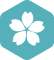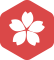### 散点 ~zell419[转] Matlab 将图像绘制成3维立体散点

Matlab

import matplotlib.pyplot as plt import numpy as np # 保证图片在浏览器内正常显示 import time start=time.time() # 10个点 N = 10 x = ['Sur', "Fea", "Dis", "Hap", "Sad", "Ang", "Nat", "XXX", "YYY", "ZZZ"]...

R语言绘图-散点

R语言绘图-散点散点图是展示两个或多个数值型变量相关性特征的常用工具，通常包括简单散点图、三维散点图、矩阵散点图等。简单散点图简单散点图是将观测数据绘制在一个二维平面中，以此观察数值型变量之间的相关性...

Matlab R2017b 绘制散点

Stata画图——散点图与折线图

Matlab由三维散点绘制三维曲面（含等高线，剖面图）

MATLAB绘制散点

scatter(X,Y,size,color)在向量X和Y的指定位置绘制彩色的圆圈。很显然X与Y的大小是必须相同的。...scatter函数可以在后面再加两个参数如scatter(x,y,6,z)其中6为散点的大小，z为散点样式。 绘制三维的散...

R中散点

MATLAB~~~描绘散点图函数scatter

X和Y是数据向量，以X中数据为横坐标，以Y中数据位纵坐标描绘散点图，点的形状默认使用圈。 &gt;&gt; x=[7 8 9 4 5 6 ] x =  7 8 9 4 5 6 &gt;&gt; y=[85 96 74 52 56 23]; &gt;&gt; ...

Python实现散点图和散点图矩阵的绘制

matlab绘制散点图——指定散点落于一个圆形区域内

matlab绘制散点图——指定散点落于一个圆形区域内 问题： 在做海豚捕食沙丁鱼的仿真时，我们将沙丁鱼群看成了一个圆形区域内的散点，所以需要用matlab在一个圆形区域内绘制均匀分布的散点。 matlab代码实现 % ...

Python可视化-散点图与彩色散点

ggplot2-绘制散点

ggplot2 绘制散点

D3.js-散点

echarts 散点

excel散点图插件

Python--Matplotlib数据可视化教程（散点图）

MPAndroidChartSample散点

http://blog.csdn.net/qq_26787115/article/details/53258500

MATLAB——绘制散点

matlab 散点图实验数据

matplotlib——散点

import matplotlib.pyplot as plt x_values = list(range(1, 1001)) y_values = [x**2 for x in x_values] # 将两个列表都传递给scatter(),matplotlib依次从每个列表中读取一个值来绘制一个点，例如（1,1）（2,4）...

c#绘制散点

c#绘制散点

Python散点图（Pandas + Matplotlib）实战--身高体重分布图

Origin绘制散点图个性化设置散点颜色

R语言 散点

Python-画图（散点图scatter、保存savefig）及颜色大全